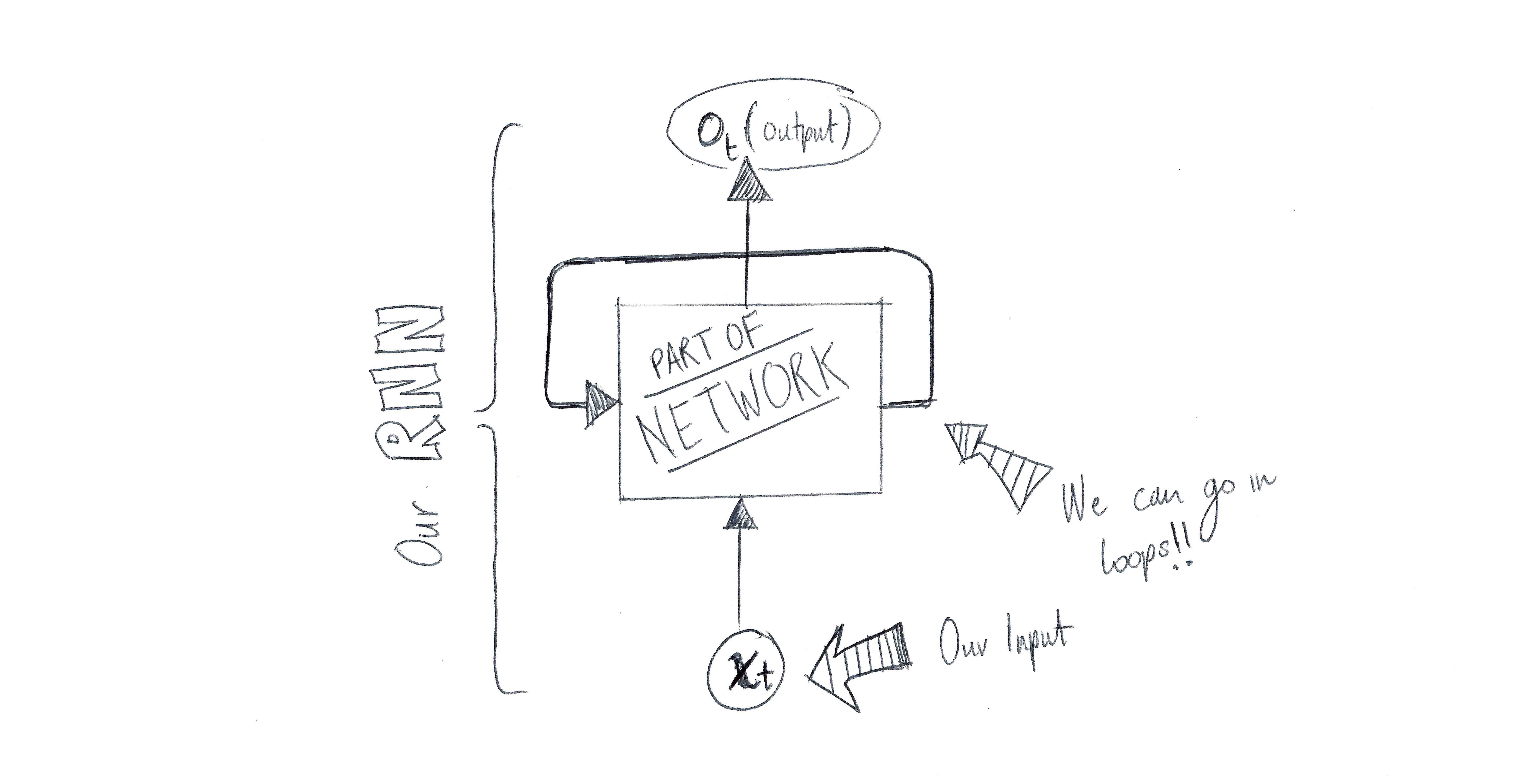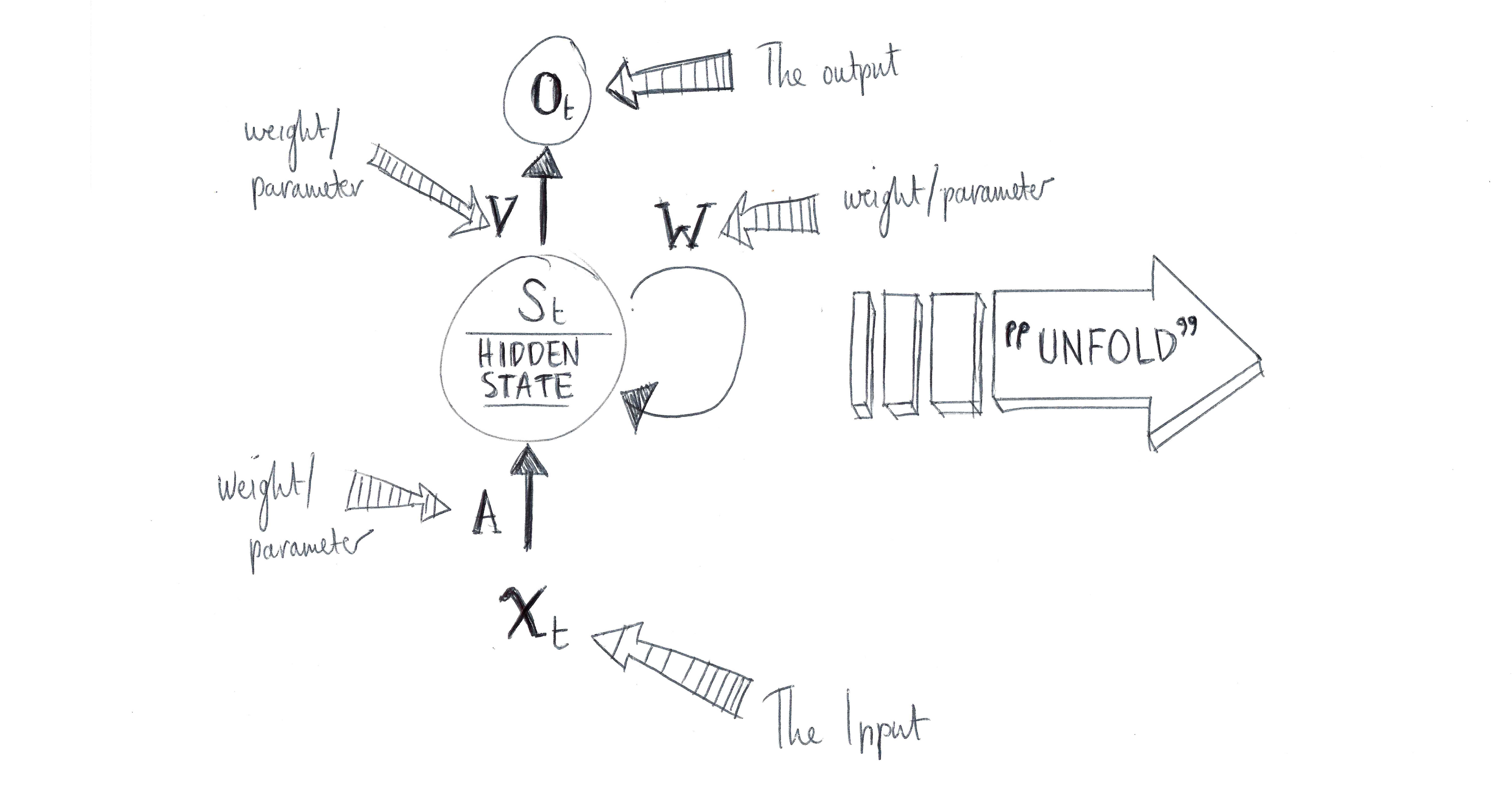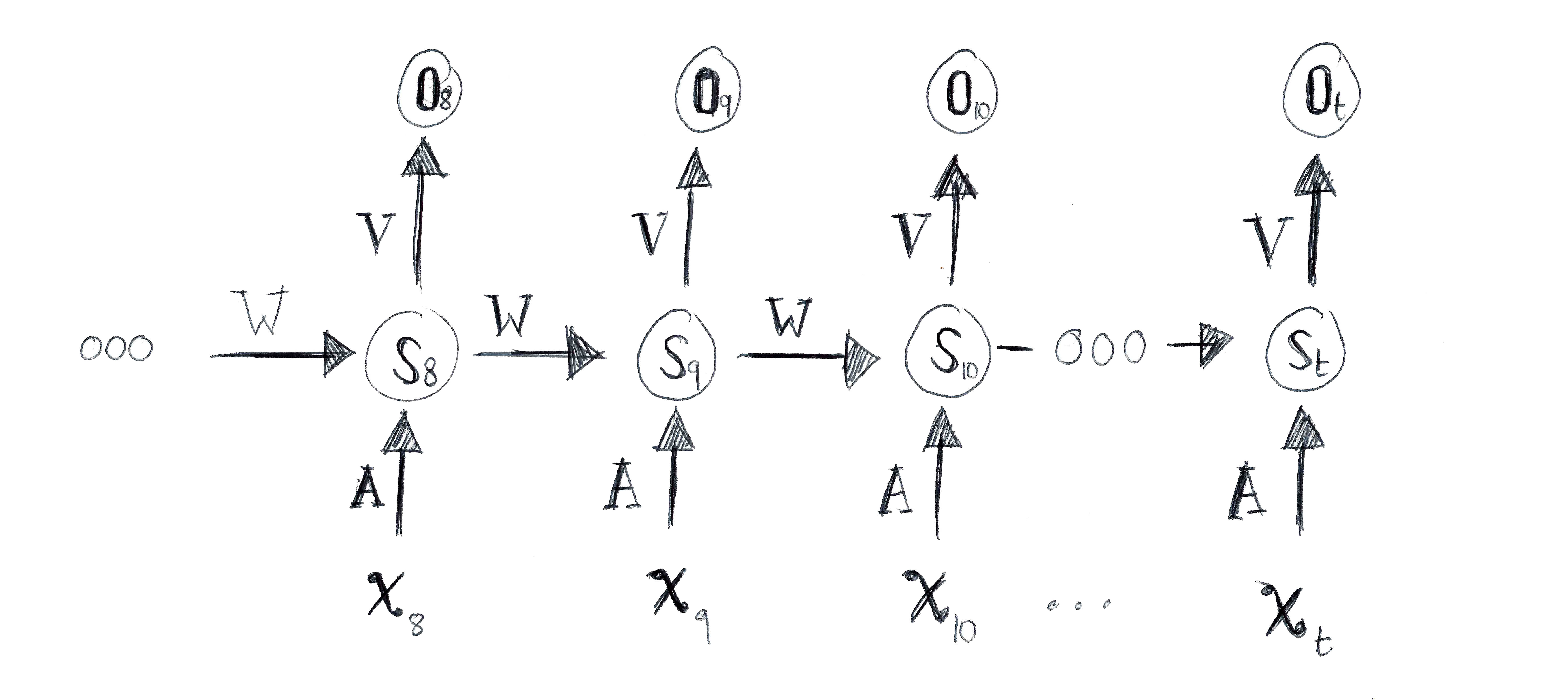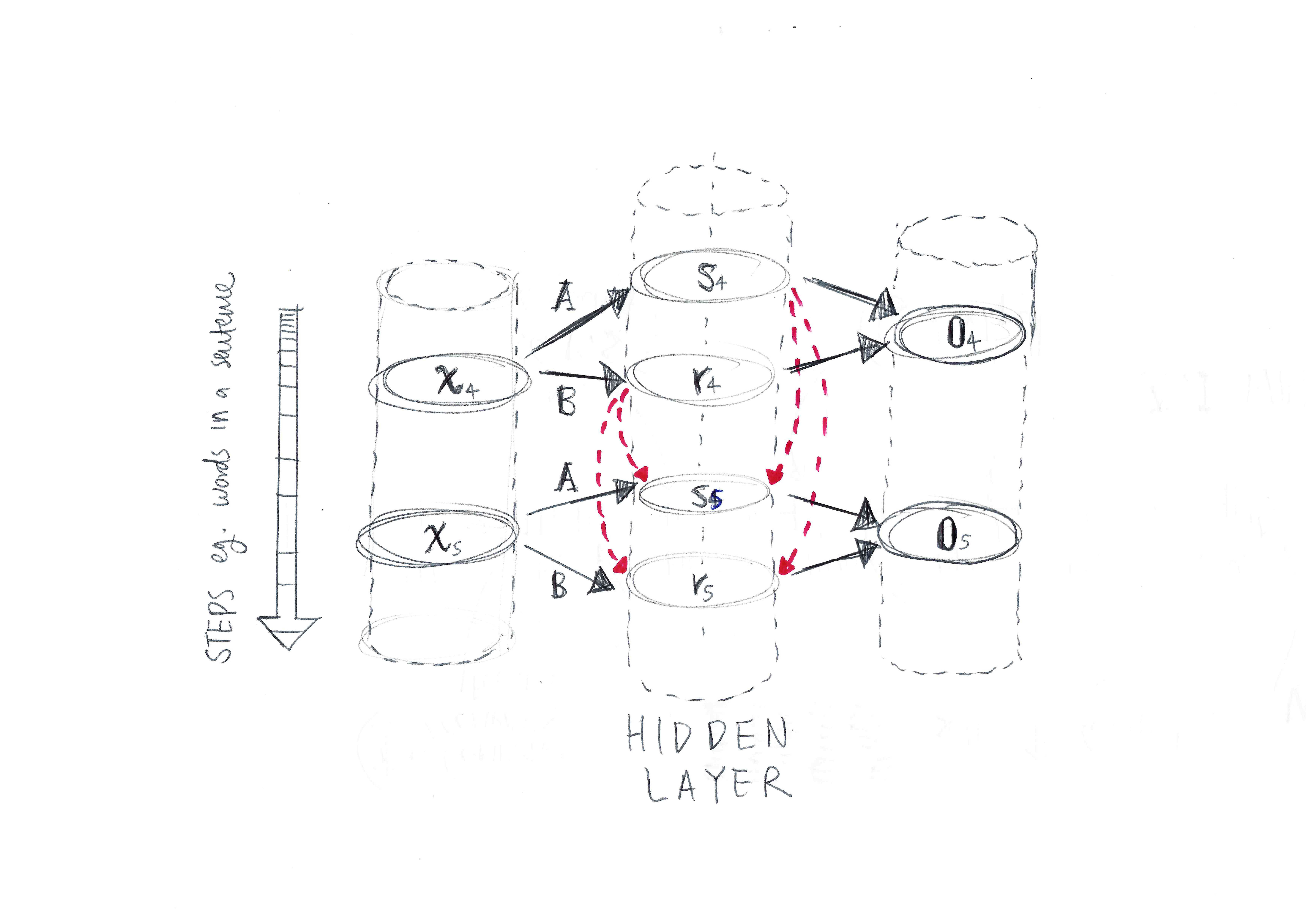Home

Themselves. Goldfish. Your.

Those are the fourth, fifth, and sixth words from the next three sentences. Sitting there by themselves that have some meaning, but you couldn’t tell me If this goldfish is dead, alive, or just a drawing. If you were a goldfish (that hypothetically speaks english), could you understand even one sentence if you kept forgetting the start by the end, let alone the last word upon hearing the next?

You absolutely couldn’t, you require your thoughts (which is information) to persist. In a traditional neural network there is no persistence. You feed something in wholesale and you get something output at the end. Information does not really persist in the network.

Recurrent Neural Networks (partially) solve this issue. The RNNs have ‘loops’ in them, which ‘pass back’ information allowing it to be used in future calculation. Wa-hey, we have memory.The above diagram is expresses simply that part of an RNN will look at some input xt and will in the process of processing that input (of t dimension/elements) will pass computation results around in the network before spitting out a result.

How does this looping work? If can imagine that each part of the input is a step, then the same RNN is ‘copied’ t number of times, with each successive step passing a ‘message’ to the its successor. Here’s the network unrolled.By unrolling we mean that we visualise each step of the network’s computing the t length input. For example, if we are running a sentiment analysis on a sentence of 9 words, the network unrolls to a 9 layer neural network. In this diagram, we can see that:

1. xt is the input at step t. This may be the word “disgustingly”, corresponding to the 4th word in the sentence. (Note that the word alone is not enough for sentiment analysis).
2. ot is the output at step t. For example in our Sentiment Analysis example it could be the positivity value (0.000 -> 1.000) of the sentence so far.
3. st is the hidden state at step t. It the bit that has the network memory persistence, as it is calculated based on the previous hidden state and the input at the current step.
4. The RNN is sharing the parameters (A,V,W) across all steps. This is because we are performing the same task at each step, just with different input and a different context.

The final sketch is a diagram showing another way of visualising the RNN, as a ‘projection’ rather than an unrolling. This diagram also shows two hidden neurons rather than one.* Drawings sketched by myself.